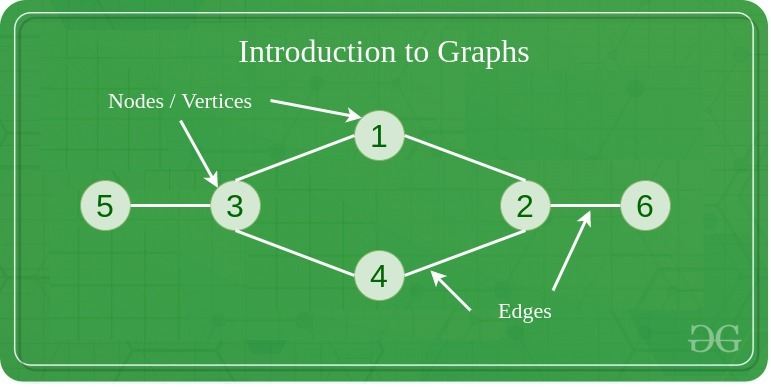GeeksforGeeks App
Open AppBrowser
Continue

# How to create a random Graph in C++?

A graph is a type of non-linear data structure that has two types of components “Vertices” (nodes) and “edges”. It contains a set of vertices (V) and a set of edges (E). Two nodes of the graph are connected by an edge. A graph is denoted by G(V, E).Example of a Graph

## Ways to represent a Graph:

There are two major ways of representing a Graph in C++:

The other way of representing the graph may include Incidence Matrix and Incidence List. The way to represent the graph we use is based on our requirements.

The graph is represented in the form of a Matrix. It is a V * V matrix, where V represents the number of vertices present in the graph.

Let us take a matrix as adj[][]. If there is any value in the cell adj[i][j], it represents that there is an edge from i to j. The adjacency matrix for an undirected graph is always symmetric. It can be used to represent the weighted graph and unweighted graph. In an unweighted graph adj[i][j] = 1 but in a weighted graph adj[i][j] is equal to the weight of the edge.It is also a common method of representing the graph. Let the list be array[]. An entry array[i] represents the list of vertices that are adjacent to the ith vertex. It can also be used to represent a weighted graph. When it is being used to represent a weighted graph then the weights of edges can be represented as lists of pairs.## Generate a random Graph using an Adjacency Matrix:

Follow the below steps to implement:

• We create a function named GenRandomGraphs and pass a variable to it. (the variable is the number of edges and vertices)
• Generate random edges till all the possible edges are covered.
• For each edge generated, check if this edge was previously generated or if both ends of the edge are the same.
• After this, traverse for each vertex:
• Find out the edges for which the current vertex is an end node.
• Insert that in the adjacency list for the current vertex.

Below is the implementation of the above approach.

## C++

 `// C++ code to implement the approach`` ` `#include ``using` `namespace` `std;`` ` `// Function to generate random graph``void` `GenRandomGraphs(``int` `NOEdge, ``int` `NOVertex)``{``    ``int` `i, j, edge[NOEdge], count;``    ``i = 0;`` ` `    ``// Assign random values to the number``    ``// of vertex and edges of the graph,``    ``// Using rand().``    ``while` `(i < NOEdge) {``        ``edge[i] = ``rand``() % NOVertex + 1;``        ``edge[i] = ``rand``() % NOVertex + 1;`` ` `        ``// Print the connections of each``        ``// vertex, irrespective of the``        ``// direction.``        ``if` `(edge[i] == edge[i])``            ``continue``;``        ``else` `{``            ``for` `(j = 0; j < i; j++) {``                ``if` `((edge[i] == edge[j]``                     ``&& edge[i] == edge[j])``                    ``|| (edge[i] == edge[j]``                        ``&& edge[i] == edge[j]))``                    ``i--;``            ``}``        ``}``        ``i++;``    ``}``    ``cout << ``"The generated random graph is: "` `<< endl;``    ``for` `(i = 0; i < NOVertex; i++) {``        ``count = 0;``        ``cout << ``"\t"` `<< i + 1 << ``"-> { "``;``        ``for` `(j = 0; j < NOEdge; j++) {``            ``if` `(edge[j] == i + 1) {``                ``cout << edge[j] << ``" "``;``                ``count++;``            ``}``            ``else` `if` `(edge[j] == i + 1) {``                ``cout << edge[j] << ``" "``;``                ``count++;``            ``}``            ``else` `if` `(j == NOEdge - 1 && count == 0)`` ` `                ``// Print “Isolated vertex”``                ``// for the vertex having``                ``// no degree.``                ``cout << ``"Isolated Vertex!"``;``        ``}``        ``cout << ``" }"` `<< endl;``    ``}``}`` ` `// Driver code``int` `main()``{``    ``int` `i, e, n;``    ``cout << ``"Random graph generation: "` `<< endl;`` ` `    ``n = 7 + ``rand``() % 6;``    ``cout << ``"The graph has "` `<< n << ``" vertices"` `<< endl;`` ` `    ``e = ``rand``() % ((n * (n - 1)) / 2);``    ``cout << ``"and has "` `<< e << ``" edges."` `<< endl;`` ` `    ``// Function call``    ``GenRandomGraphs(e, n);`` ` `    ``return` `0;``}`

Output

```Random graph generation:
The graph has 8 vertices
and has 18 edges.
The generated random graph is:
1-> { 5 4 2  }
2-> { 4 8 6 3 1 5  }
3-> { 5 4 7 2  }
4-> { 2 3 7 1 8 5  }
5-> { 3 1 7 4 2 8  }
6-> { 2 8 7  }
7-> { 4 3 5 6  }
8-> { 2 6 4 5  }```

Time Complexity: O(E2 + E*V) where E is the number of edges and V is the number of vertices
Auxiliary Space: O(V2)

Related Articles:

My Personal Notes arrow_drop_up